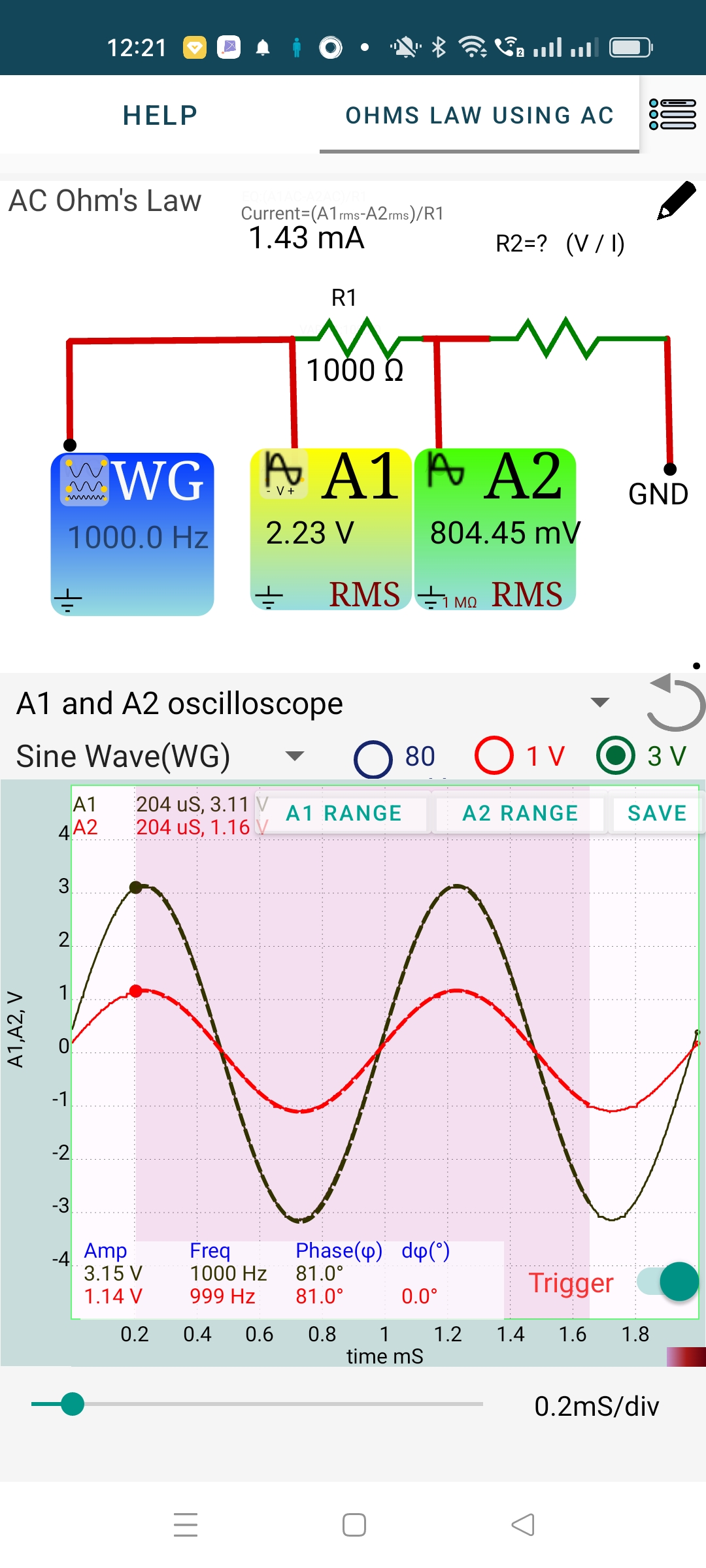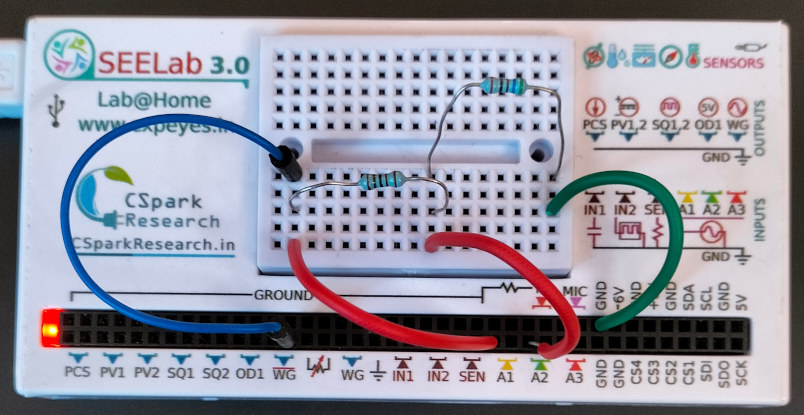# ExpEYES

## Study of Ohm's law using AC

The relationship between voltage, current and resistance is given by Ohm’s Law. It states that Resistance = Voltage across the conductor / current though it. $R = \frac{V}{I}$ This has been explored using a DC voltage earlier. Now we will repeat it using an AC source.

A 1000 Ohm resistor (R1) is connected between WG and A1. Another resistor (R2) of unknown value is connected from A1 to Ground. The current through R1 is given by $\frac{WG}{A1}, both are known. We assume that the same current flows through R1 and R2. The finite input impedance of A1 ($ 1M\omega \$) implies that some amount of current flow into it, but itcan be neglected while working with resistors below 10 KOhm.

The RMS value of the AC voltage is displayed. You need to select a region on the graph to get the voltage displayed on the WG and A1 icons. The current through R1 also will be displayed. The calculations are similar to that of DC.

From the screenshot shown below:

• Voltage across R2 = 0.804 V
• Current = 1.43 mA
• R = .804/0.00143 = 562 Ohms

Repeat it using several other values.